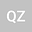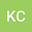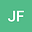loading page

Existence of axially symmetric solutions for a kind of planar Schr\”{o}dinger-Poisson system
•••• +1Kai Chen
Guilin University of Aerospace Technology
Author ProfileJinmei Fan
Guilin University of Technology
Author ProfileShuqin Liu
Guilin University of Technology
Author Profile## Abstract

In this paper, we study the following kind of Schr\”{o}dinger-Poisson system in ${\R}^{2}$ \begin{equation*} \left\{\begin{array}{ll} -\Delta u+V(x)u+\phi u=K(x)f(u),\ \ \ x\in{\R}^{2},\\ -\Delta \phi=u^{2},\ \ \ \ \ \ \ \ \ \ \ \ \ \ \ \ \ \ \ \ \ \ \ \ \ \ \ \ \ \ \ \ x\in{\R}^{2}, \end{array}\right. \end{equation*} where $f\in C({\R}, {\R} )$, $V(x)$ and $K(x)$ are both axially symmetric functions. By constructing a new variational framework and using some new analytic techniques, we obtain an axially symmetric solution for the above planar system. our result improves and extends the existing works.